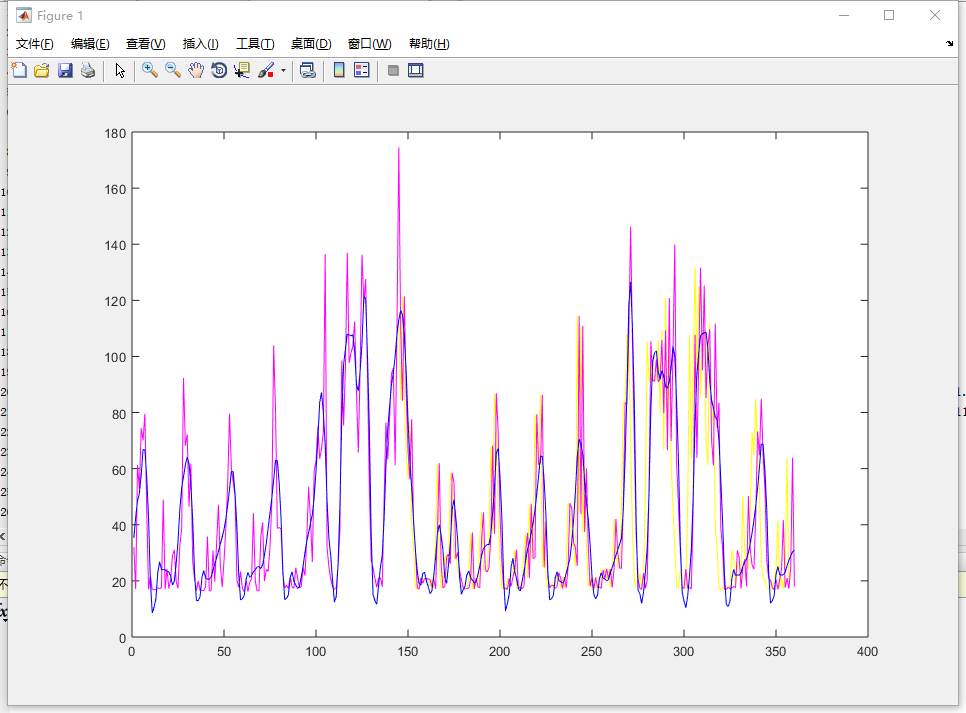β

# 基于小波变换的时间序列降噪

### 0x01 小波去噪的基本原理

1. 选择合适的正交小波基和分解层数j，对含噪信号进行小波变换分解到j层；

2. 对分解得到的小波系数进行阈值处理，可以使用两种处理方法：硬阈值和软阈值法。

### 0x02 Matlab 实现一维小波降噪

• wavedec: 一维信号的多尺度分解，将返回诸多细节系数和每个系数的长度;
• waverec: 一维信号小波分解系数的重构，将返回重构后的信号;
• wdencmp: 对一维或二维信号的压缩或者去噪;Matlab代码

``````clc;
clear;
% 获取噪声信号
S=importdata('data_0391');
sig = S(1:1400);

% 信号的分解
[c,l]=wavedec(sig,4,'db4');

%提取四层细节分量和近似分量
a1=appcoef(c,l,'db4',1);
d1=detcoef(c,l,1);
a2=appcoef(c,l,'db4',2);
d2=detcoef(c,l,2);
a3=appcoef(c,l,'db4',3);
d3=detcoef(c,l,3);
a4=appcoef(c,l,'db4',4);
d4=detcoef(c,l,4);

% 重构小波分解向量，其中第一、二层的细节分量被置零
dd1=zeros(size(d1));
dd2=zeros(size(d2));
c1=[a4 d4 d3 dd2 dd1];
aa1=waverec(c1,l,'db4');

% 作图
subplot(211),
plot(sig), title('原始噪声信号');
plot(212)
plot(aa1,'b')
title('1&2层的细节分量置零后的重构信号')
``````

### 0x03 Python 实现一维小波降噪

pyWavelet 的主要特点：

1. 类似 Matlab Wavelet Toolbox 的使用；
2. 1D, 2D 正反离散小波变换；
3. 70 多个内置小波滤波器。

Python代码

``````import pywt

# 小波滤噪
def wavelet_denoising(data):
# 小波函数取db4
db4 = pywt.Wavelet('db4')
if type(data) is not types.NoneType:
# 分解
coeffs = pywt.wavedec(data, db4)
# 高频系数置零
coeffs[len(coeffs)-1] *= 0
coeffs[len(coeffs)-2] *= 0
# 重构
meta = pywt.waverec(coeffs, db4)
return meta
``````